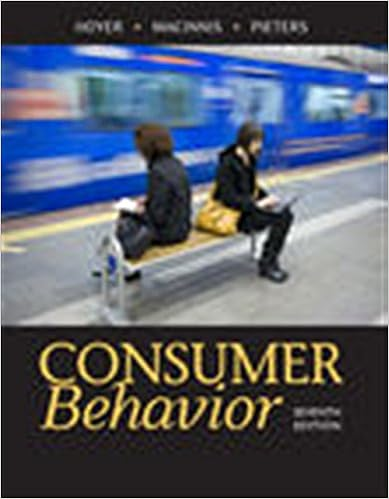# If a products income elasticity of demand is 17 then

• 35
• 88% (8) 7 out of 8 people found this document helpful

This preview shows page 5 - 7 out of 35 pages.

##### We have textbook solutions for you!
The document you are viewing contains questions related to this textbook.The document you are viewing contains questions related to this textbook.
Chapter 15 / Exercise 8
Consumer Behavior
Hoyer/MacInnisExpert Verified
9) If a product's income elasticity of demand is -1.7, then we can conclude that A) an increase in income will lead to an increase in demand for the product. B) the product is certainly a necessity. C) the product is a luxury good. D) a decrease in income will lead to an increase in demand for the product. E) the product is a normal good.
10) Normal goods
Figure 1: The initial market equilibrium is P = \$2.00 and Q = 1000. Suppose the government imposes a tax of \$0.60 per soft drink purchased. 11) Refer to Figure 1. After the tax is imposed, the price paid by the consumer becomes
12) Refer to Figure 1. The after-tax price received by the seller becomes
13) Refer to Figure 1. After the tax is imposes, the change in total expenditure on soft drinks is A) a decrease of \$80. B) a decrease of \$160. C) an increase of \$320. D) an increase of \$480. E) No change in total expenditure.
##### We have textbook solutions for you!
The document you are viewing contains questions related to this textbook.The document you are viewing contains questions related to this textbook.
Chapter 15 / Exercise 8
Consumer Behavior
Hoyer/MacInnisExpert Verified
14) The imposition of an excise tax will cause the least burden on consumers when demand is
15) A value of zero for the elasticity of supply of some product implies that
16) If pizza and beer are complementary goods, we can conclude that
17) Suppose the cross-price elasticity of demand between raspberry jam and strawberry jam is 7.5. The interpretation of this result is that A) a 10% increase in the price of strawberry jam leads to a 7.5% increase in quantity demanded of raspberry jam. B) a 10% increase in the price of strawberry jam leads to a 0.75% increase in quantity demanded of raspberry jam. C) a 10% increase in the price of strawberry jam leads to a 75% increase in quantity demanded of raspberry jam. D) a 10% increase in the price of strawberry jam leads to a 7.5% decrease in quantity demanded of raspberry jam. E) a 10% increase in the price of strawberry jam leads to a 75% decrease in quantity demanded of raspberry jam.
•••